﻿ NCTS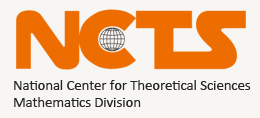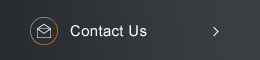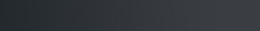Sponsored by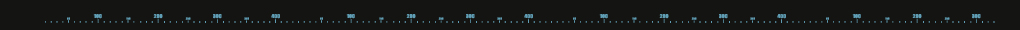[ Events ]

﻿
Activity Search
Sort out
 select all Seminars and Talks Conference and Workshop Lectures and Courses Else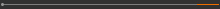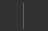Field
 select all Number Theory and Representation Theory Algebraic Geometry Differential Geometry and Geometrical Analysis Differential Equations and Stochastic Analysis Scientific Computing Interdisciplinary Studies Laboratory of Data Science Harmonic Analysis OthersYear
 select 2015 2016 2017 2018 2019 2020 2021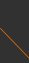Taiwan Mathematics School: Statistical Foundations of Medical AI: Linear RegressionEvery Thursday, 13:20-16:20, September 17 - October 22, 2020 R. 215, College of Public Health, NTU Speaker: Hung Hung (National Taiwan University) Organizers: Weichung Wang (National Taiwan University) I. Course Goal： This course covers some of the statistical foundations for medical AI. In particular, the topics include linear regression, logistic regression, ROC curve analysis, Poisson regression, and survival analysis. The course will start from continuous data to illustrate the rationale of the linear regression model and its applications. The concept will be extended to different data structures, including binary data, count data, and survival data. Another topic of this course will focus on the implementation of the R software and the ability to analyze the real data sets. We expect students can choose the appropriate methods (as well as understand the rationale of the methods) to conduct the statistical analysis for a given data set correctly.   II. Contents： Review basic statistical analysis Simple linear regression Least squares estimate R-square & adjusted R-square mean response, prediction, residual Multiple linear regression Full & Reduced model, ANOVA Introduction of generalized linear model, MLE Logistic regression ROC curve analysis Poisson regression Introduction of survival analysis Kaplan-Meier estimator Log rank test Cox PH model and its extensions Contact: murphyyu@ncts.ntu.edu.tw(C) 2019 National Center for Theoretical Sciences